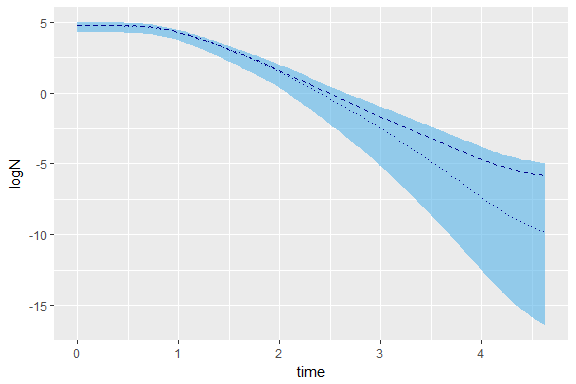# bioinactivation: Software for modelling microbial inactivation

## Introduction

R-package bioinactivation implements functions for the modelization of microbial inactivation. These functions are based on published inactivation models commonly used both in academy and industry. bioinactivation can be downloaded from CRAN. Once installed, the package is loaded with the command.

library(bioinactivation)

The bioinactivation package allows to predict the inactivation process of a population of microorganisms exposed to isothermal conditions (temperature remains constant with time) or non isothermal (temperature changes with time, dynamic conditions). Moreover, it can be used to adjust the parameters of different inactivation models to both isothermal and dynamic experimental data. All the functions contained in bioinactivation are written in R.

bioinactivation takes advantage of the capabilities of the packages deSolve (Soetaert, Petzoldt, and Setzer 2010a) and FME (Soetaert, Petzoldt, and Setzer 2010b) to make predictions of the inactivation process and to fit model parameters to experimental data. The inactivation models most commonly used in the industry have been implemented in this package:

• Bigelow model (Bigelow, 1921),
• Weibull-Peleg model (Peleg and Cole, 1998),
• Weibull-Mafart model (Mafart et al., 2002),
• Geeraerd’s model (Geeraerd et al., 2005).
• Arrhenius model (Box, 1960).

The bioinactivation package provides five functions for the modelization of microbial inactivation:

• predict_inactivation() predicts the inactivation process of a population for some given temperature conditions, isothermal or dynamic.
• fit_isothermal_inactivation() adjusts the parameters of a selected inactivation model to a set of isothermal inactivation experiments.
• fit_dynamic_inactivation() fits the parameters of a chosen inactivation model to a set of dynamic inactivation experiments using non-linear regression.
• fit_inactivation_MCMC() adjusts an inactivation model to a set of dynamic inactivation experiments using a Markov Chain Monte Carlo algorithm.
• predict_inactivation_MCMC() generates prediction intervals for isothermal or non-isothermal inactivation processes using a Monte Carlo method.

Moreover, two functions with meta information of the inactivation models implemented are included:

• get_model_data(), provides meta information about the models used for prediction and adjustment of non-isothermal experiments.

• get_isothermal_model_data(), provides meta information about the models for fitting of isothermal experiments.

Furthermore, four data sets mimicking the results of an isothermal inactivation experiment (isothermal_inactivation and laterosporus_iso) and a dynamic experiment (dynamic_inactivation and laterosporus_dyna) are included in the package.

bioinactivation does not require the user to introduce the data in any particular units system. The units system used for input is kept on the output. For this reason, units are not shown in any figure within this document.

## Example data sets included in the package

### The example data set of an isothermal experiment

The bioinactivation package includes the isothermal_inactivation data set, which imitates the data obtained during a set of isothermal inactivation experiments. This data set can be loaded as:

data(isothermal_inactivation)

This data set supplies experiments made at six different temperature levels, with a variable number of observations per experiment. In total, the data set contains 68 observations of 3 variables:

• The first variable (time) provides the experiment time at which the observation was made.

• The second column (temp) contains the temperature of the experiment at a given time.

• The logarithmic difference between the initial concentration and the one at a time-temperature combination is given by the third column (log_diff).

The following plot illustrates the data contained in the data set.

library(ggplot2)
ggplot(data = isothermal_inactivation) +
geom_point(aes(x = time, y = log_diff, col = as.factor(temp))) +
ggtitle("Example dataset: isothermal_inactivation")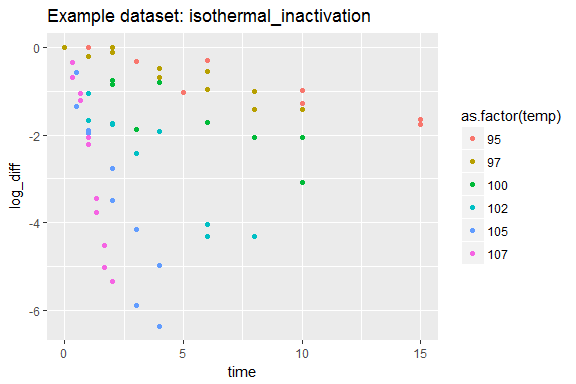### The example data set of a dynamic experiment

The dynamic_inactivation data set contained within the bioinactivation package provides a data set imitating the data obtained during a non-isothermal inactivation experiment. This data set can be loaded as:

data(dynamic_inactivation)

This example data set contains 3 variables and 19 observations. The first column, time, reflects the time point at which the observation was taken. The second and third ones provide the number of microorganism (N) and the temperature (temperature) recorded.

The following plots depicts the data contained in the data set.

ggplot(data = dynamic_inactivation) +
geom_point(aes(x = time, y = log10(N))) +
ggtitle("Example dataset: dynamic_inactivation. Observations")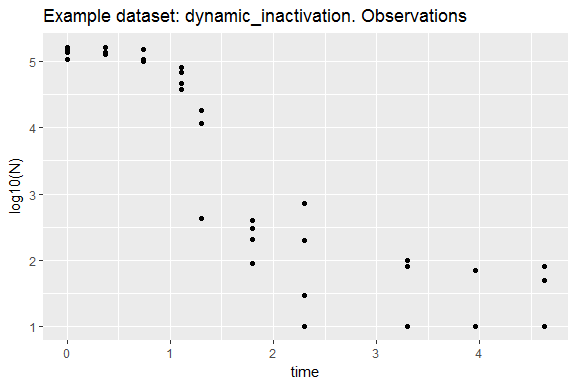ggplot(data = dynamic_inactivation) +
geom_point(aes(x = time, y = temperature))  +
ggtitle("Example dataset: dynamic_inactivation. Temperature profile")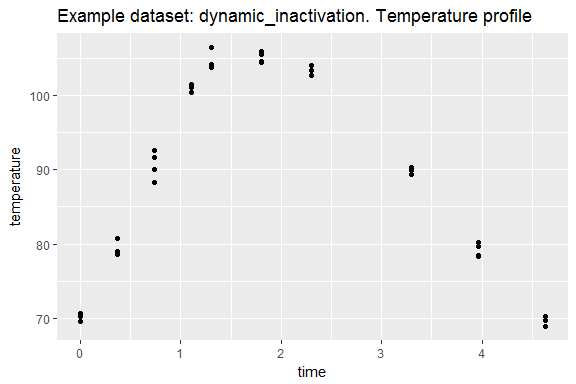### The example data set of an isothermal study of Bacillus laterosporus

bioinactivation contains a data set with the isothermal inactivation observed in a population of B. laterosporus. This data set can be loaded as:

data(laterosporus_iso)

This data set contains 52 observations and 3 different variables:

• The first variable (time) provides the experiment time at which the observation was made.
• The second column (temp) contains the temperature of the experiment at a given time.
• The logarithmic difference between the initial concentration and the one at a time-temperature combination is given by the third column (log_diff).

The following plot illustrates the data contained in the data set.

ggplot(data = laterosporus_iso) +
geom_point(aes(x = time, y = log_diff, col = as.factor(temp))) +
ggtitle("Example dataset: laterosporus_iso")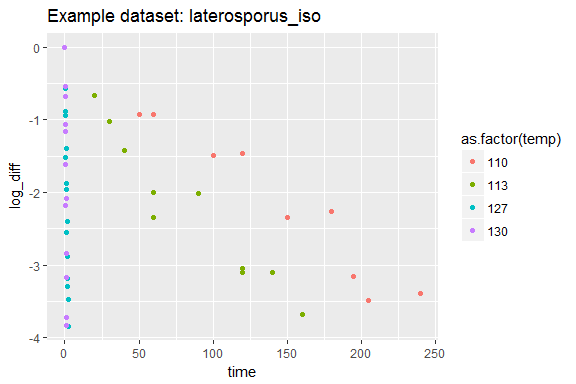### The example data set of a non-isothermal study of laterosporus

The data set laterosporus_dyna included in bioinactivation contains the results of a series of non-isothermals studies performed on a population of B. laterosporus. This data set can be loaded as:

data(laterosporus_dyna)

This data set contains 20 observations with 3 variables:

• time includes the time spent betwen the beginning of the experiment and the measurement.
• temp is the temperature recorded at the moment of the observation.
• logN is the logarithmic number of microorganism observed.

The following plots depict the data within the data set.

ggplot(data = laterosporus_dyna) +
geom_point(aes(x = time, y = logN)) +
ggtitle("Example dataset: laterosporus_dyna. Observations")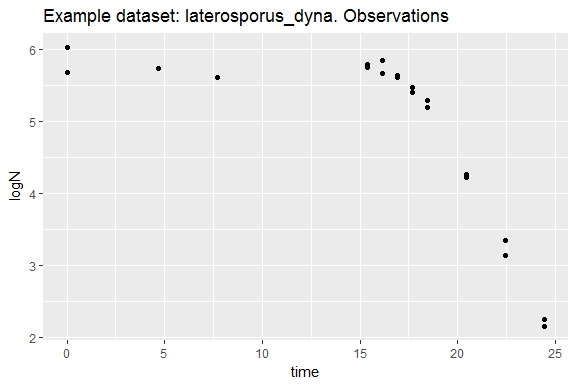ggplot(data = laterosporus_dyna) +
geom_point(aes(x = time, y = temp))  +
ggtitle("Example dataset: laterosporus_dyna. Temperature profile")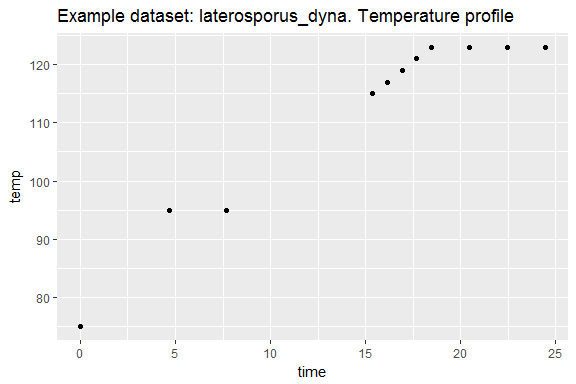## Inactivation models implemented

The inactivation models most commonly used in both academy and industry for the modelization of microbial inactivation have been implemented in the bioinactivation package:

• Bigelow model (Bigelow, 1921),
• Weibull-Peleg model (Peleg and Cole, 1998),
• Weibull-Mafart model (Mafart et al., 2002),
• Geeraerd model (Geeraerd et al., 2005).
• Arrhenius model (Box, 1960).

A brief mathematical description of each one of them is presented through this section.

### Bigelow model for isothermal data

Bigelow’s model (Bigelow, 1921) assumes that the inactivation process follows a first order kinetics. Therefore, a linear relation exists between the logarithm of the number of microorganisms ($$N$$) and the time ($$t$$).

$\mathrm{log}_{10}(N) = \mathrm{log}_{10}(N_0) - \frac{1}{D(T)}t$

The parameter $$D(T)$$ (also named D-value) equals the negative inverse of the slope of the line. Its relationship with temperature ($$T$$) is described by the equation

$\mathrm{log}_{10}D(T) = \mathrm{log}_{10}D_R + \frac{T_R-T}{z}$

where $$T_R$$ is a reference temperature and $$D_R$$ the D-value at this temperature. The parameter $$z$$ is usually named z-value and provides an indication of the sensitivity of the microorganism to temperature variations.

### Bigelow model for nonisothermal data

Bigelow’s model for nonisothermal data is developed from the isothermal one by calculating the first derivative of its constitutive equation with respect to time.

$\frac{d\mathrm{log}_{10}(N)}{dt} = - \frac{1}{D(T)}$

In this model, the evolution of $$D(T)$$ with temperature is ruled by the expression used for the isothermal model:

$\mathrm{log}_{10}D(T) = \mathrm{log}_{10}D_R + \frac{T_R-T}{z}$

### Weibullian models

Weibullian models assume that the the inactivation process can be viewed as a failure phenomenon. According to this hypothesis, the time that each microorganism within the population can sustain some environmental conditions follows a Weibull-type probability distribution. Two models of this family have been implemented: Weibull-Peleg (Peleg and Cole, 1998) and Weibull-Mafart (Mafart et al., 2002).

#### Weibull-Peleg model for isothermal inactivation

The Weibullian model presented by Peleg and Cole (1998) for isothermal cases follows the equation:

$\log_{10}S(t) = - b \cdot t^n$

where $$S = N/N_0$$ represents the fraction of survivors and $$t$$ the time, and $$b$$ and $$n$$ are the two parameters of the model.

In the Weibull-Peleg model the parameter $$n$$ is assumed to be temperature independent, while the dependency of $$b$$ with temperature is modeled as

$b(T) = \mathrm{ln} \big\{ 1 + \mathrm{exp} \big[ k_b(T - T_c) \big] \big\}$

This equation is based on the definition of a critical temperature ($$T_c$$). For temperatures ($$T$$) much lower than $$T_c$$, $$b(T) \simeq 0$$ and the inactivation is insignificant. For temperatures close to $$T_c$$, there is an exponential growth of $$b(T)$$ with respect to the temperature. For temperatures much higher than the critical one, a linear relation exists between $$T$$ and $$b(T)$$ with a slope $$k_b$$. This evolution is illustrated in the following plot: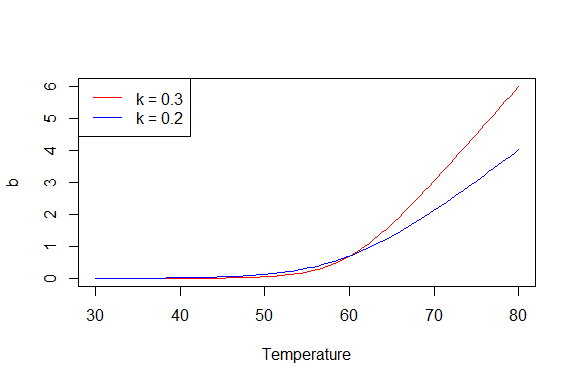#### Weibull-Peleg model for non-isothermal inactivation

The model described by Peleg and Cole (1998) for dynamic inactivation has been implemented in the bioinactivation package. This model is based on the constitutive equation of the Weibull-Peleg model (Peleg and Cole, 1998) for isothermal inactivation:

$\log_{10}S(t) = -b(T) \cdot t^n$

The inactivation rate for a constant temperature can be calculated as the first derivative with respect to time of this equation:

$\Bigg| \frac{d \log_{10}S(t)}{dt} \Bigg|_{T=const} = -b(T) \cdot n \cdot t^{n-1}$

The time required to achieve any survival ratio, $$t^\star$$, can be calculated from the model equation as:

$t^\star = \Bigg| \frac{-\mathrm{log}_{10}S(t)}{b(T)} \Bigg|^{1/n}$

Substituting this expression into the rate equation, the differential constitutive equation of the model is obtained as:

$\Bigg| \frac{d \log_{10}S(t)}{dt} \Bigg| = -b(T) \cdot n \cdot \Bigg| \frac{ -\mathrm{log}_{10}S(t) }{b(T)} \Bigg|^\frac{n-1}{n}$

where $$b(T)$$ follows the same expression as for the isothermal model:

$b(T) = \mathrm{ln} \big\{ 1 + \mathrm{exp} \big[ k(T - T_c) \big] \big\}$

#### Weibull-Mafart model for isothermal inactivation

The Weibullian model presented by Mafart et al. (2002) for isothermal cases is described by the equation:

$\mathrm{log}_{10}S(t) = - \Big( \frac{t}{\delta(T)} \Big)^\beta$

where $$S = N/N_0$$ represents the fraction of survivors and $$t$$ is the time. $$\delta$$ and $$\beta$$ are, respectively, the rate and shape factors of the Weibull distribution.

In this model, $$\beta$$ is considered to be temperature independent and $$\delta$$ follows and exponential relation with temperature ($$T$$):

$\delta(T) = \frac {\delta_{ref}} {10^ {\big( T-T_{ref}/z \big)} }$

where $$T_{ref}$$ is a reference temperature, $$\delta_{ref}$$ is the value of $$\delta$$ at this reference temperature and $$z$$ describes the sensitivity of the microorganism to temperature variations.

#### Weibull-Mafart model for nonisothermal inactivation

The dynamic Weibull-Mafart model is developed from the isothermal one. The isothermal rate of logarithmic reduction at each time is calculated as the first derivative of the constitutive equation with respect to time:

$\frac{d\mathrm{log}_{10}S(t)}{dt} = -\beta \frac{t^{\beta-1}}{\delta(T)^\beta}$

As well as for the isothermal model, an exponential relation between $$\delta(T)$$ and temperature is assumed:

$\delta(T) = \frac {\delta_{ref}} {10^ {\big( T-T_{ref}/z \big)} }$

### Geeraerd model

The inactivation model described by Geeraerd et al. (2005) is based on the assumption that the inactivation process follows a first order kinetics with rate $$k$$.

$\frac {dN}{dt} = - k \cdot N$

However, the parameter $$k$$ is corrected in this model with two parameters to include for shoulder ($$\alpha$$) and tail ($$\gamma$$) effects:

$k = k_{max} \cdot \alpha \cdot \gamma$

where $$k_{max}$$ stands for the maximum inactivation rate of the microorganism at the current environmental conditions.

A secondary model equivalent to Bigelow’s is used to describe the evolution of $$k_{max}$$ with temperature ($$T$$):

$k_{max} = \frac{1}{D(T)}$ $\mathrm{log}_{10}D(T) = \mathrm{log}_{10}D_R + \frac{T_R-T}{z}$

where $$D(T)$$, $$T_R$$ and $$z$$ are the D-value, reference temperature and z-value respectively.

The shoulder parameter is defined as

$\alpha = \frac {1}{1+C_c}$

where $$C$$ is a dummy component that limits the microbial inactivation. It is assumed that this component also follows a first order kinetics with rate $$k_{max}$$:

$\frac {dC_c}{dt} = - k_{max} \cdot C_c$

The tail parameter is defined by the equation

$\gamma = 1 - \frac{N_{res}}{N}$

where $$N_{res}$$ stands for the residual number of microorganism after the treatment.

### Arrhenius inactivation model

Several versions of the Arrhenius model are available in the literature. In bioinactivation, a log-linear primary inactivation model:

$\frac{ dN}{dt} = -k(T)\cdot N$

where $$k(T)$$ represents the specific inactivation rate at temperature ($$T$$). The reparametrization proposed by Box (1960) was used as secondary model:

$k(T) = k_{ref} \exp{ \left[- \frac{E_a}{R} \left( \frac{1}{T} - \frac{1}{T_{ref}} \right) \right]}$

where $$k_{ref}$$ is the value of $$k$$ at the reference temperature $$T_{ref}$$. $$E_a$$ is the activation energy and $$R = 8.31 J\cdot mol^{-1} K^{-1}$$, is the universal constant gas.

## Prediction of microbial inactivation

The bioinactivation package includes capabilities for the prediction of the inactivation process of a microorganism under either isothermal or dynamic conditions. This is accomplished through the predict_inactivation() function, which solves the differential equation of the model using the ode function from the deSolve package (Soetaert, Petzoldt, and Setzer 2010).

predict_inactivation() requires five input arguments:

• simulation_model,
• times,
• parms,
• temp_profile,
• ... and
• tol0 (optional).

simulation_model is a string identifying the model to be used. A list of the available models can be obtained by calling the function get_model_data() without any argument.

get_model_data()
##  "Bigelow"   "Mafart"    "Geeraerd"  "Peleg"     "Arrhenius"

In this example Geeraerd’s model will be used, so the value Geeraerd will be assigned.

example_model <- "Geeraerd"

times is a numeric vector which specifies the values of time when results will be returned. It does not define the points at which the numerical integration is done, so changing it does not affect the values calculated at individual time points. An arbitrary equally spaced vector will be used for this example.

times <- seq(0, 5, length=100)

parms is a named numeric vector specifying the values of the model parameters, as well as the initial values of the model variables. A call to the function get_model_data() with a valid model identifier as an input argument provides several data concerning this model as a list. Namely, its parameters and variables.

model_data <- get_model_data(example_model)
print(model_data$parameters) ##  "D_R" "z" "N_min" "temp_ref" print(model_data$variables)
##  "N"   "C_c"

The input argument parms must provide values for every degree of freedom of the model, i.e. model parameters and variables. The names of the model parameters must be identical to those provided by the function get_model_data(). On the other hand, the names assigned to the initial values of the model variables must be equal to the ones provided by get_model_data() plus a character "0". For instance, the initial value for the variable named N needs to be labeled "N0".

model_parms <- c(D_R = 1,
z = 10,
N_min = 1e2,
temp_ref = 100,
N0 = 1e5,
C_c0 = 1e1
)

The last input variable required for the function is a data frame defining a discrete temperature profile. Values of the temperature at time points not provided will be approximated by linear interpolation. In case a temperature value outside the time range specified is requested, the temperature of the closest extreme will be used.

Predictions for isothermal conditions can also be calculated using this function. In this case, a constant temperature profile has to be defined.

A temperature profile with a constant slope of 10ºC/min ranging between 70 and 120ºC will be used for the prediction.

temperature_profile <- data.frame(time = c(0, 5),
temperature = c(70, 120))
plot(temperature_profile, type = "l")
title("Example temperature profile")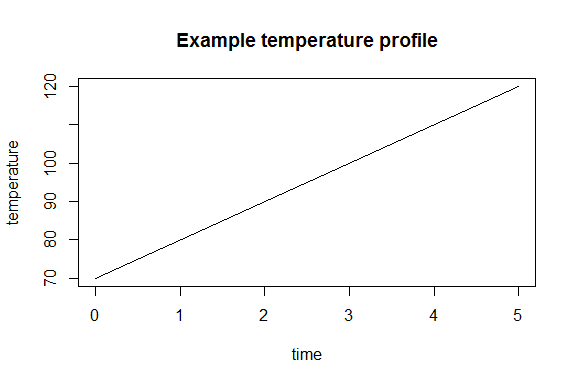The inactivation models based on the Weibull distribution are singular for $$t=0$$. For this reason, every value of time in times lower than the optional argument tol0 is changed for tol0. By default, tol0 = 1e-05.

Finally, the argument ... allows to pass adidtional arguments to the ode() function of the deSolve package.

The prediction is calculated by calling the function predict_inactivation() with the parameters described before. The optional argument will not be modified.

prediction_results <- predict_inactivation(example_model, times, model_parms, temperature_profile)

The value returned by the function is a list of class SimulInactivation. This list has four entries:

• model, a string with the identifier of the model used for the simulation.
• model_parameters, a named numeric list with the model parameters used.
• temp_approximations, a list with two entries, each providing the functions used to approximate the temperature at each time point.
• simulation a data frame with the results of the simulation.

The entry simulation provides a data frame with the calculated results.

head(prediction_results$simulation) ## time N C_c logN S logS ## 1 0.00001000 100000.00 10.000000 5.000000 1.0000000 0.000000e+00 ## 2 0.05050505 99998.88 9.998766 4.999995 0.9999888 -4.868988e-06 ## 3 0.10101010 99997.62 9.997379 4.999990 0.9999762 -1.034011e-05 ## 4 0.15151515 99996.20 9.995822 4.999984 0.9999620 -1.648678e-05 ## 5 0.20202020 99994.62 9.994075 4.999977 0.9999462 -2.338188e-05 ## 6 0.25252525 99992.83 9.992113 4.999969 0.9999283 -3.113231e-05 The first column contains the times at which results are output are given. They are equal to the values provided by the times input variable, although sorted. In case there were some repeated values in the input argument times, results are output only once. The following columns provide the results obtained for the variables of the models. In this case, as Geeraerd’s model has been used, N and C_c. The last three columns provide the values of $$\mathrm{log}_{10}(N)$$, $$S$$ and $$\mathrm{log}_{10}(S)$$. The SimulInactivation object includes an S3 method for the plotting of its results. This method is able to generate the plot using ggplot2 (default) or base R. The ggplot2 graphic can be generated by calling the plot() function with the instance of SimulInactivation as argument. The function returns an instance of ggplot which can be represented by calling the function print(). p <- plot(prediction_results) print(p)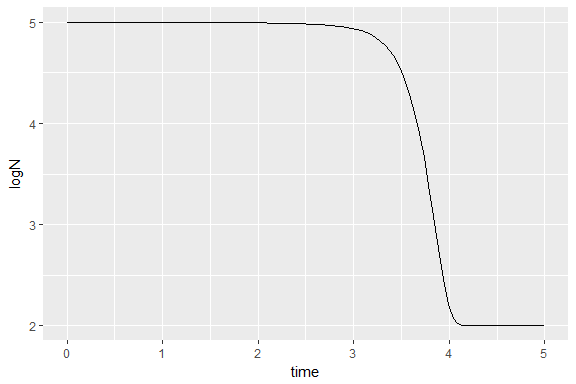Instead of directly generating the plot, the function returns the ggplot instance to ease further editting. For instance, the format and x-label can be easily modified. library(ggplot2) p + theme_light() + xlab("time (min)")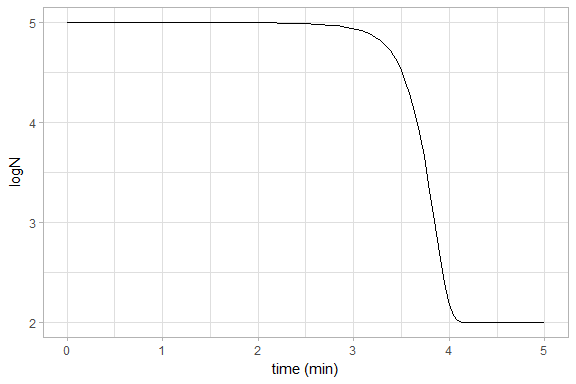Note that if the returned object is not assigned to any variable, the plot is automatically printed. On the other hand, the version of the plot in base R can be generated including the argument make_gg = FALSE in the call to plot(). plot(prediction_results, make_gg = FALSE, xlab = "Time (min)", ylab = "logN")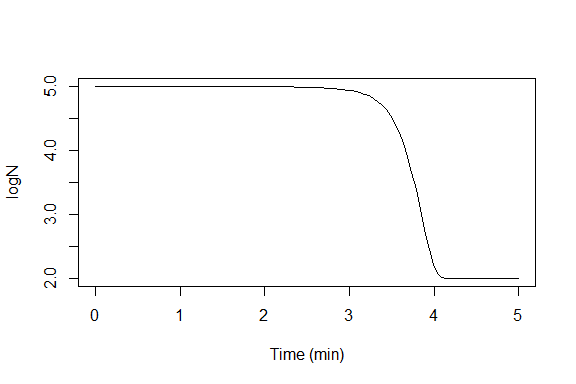The implementation of the Geeraerd model allows to model inactivation process without shoulder and/or tail effects. For the model without shoulder, assign zero to the parameter C_c0: parms_no_shoulder <- c(D_R = 1, z = 10, N_min = 100, temp_ref = 100, N0 = 100000, C_c0 = 0 ) prediction_no_shoulder <- predict_inactivation(example_model, times, parms_no_shoulder, temperature_profile) plot(prediction_no_shoulder)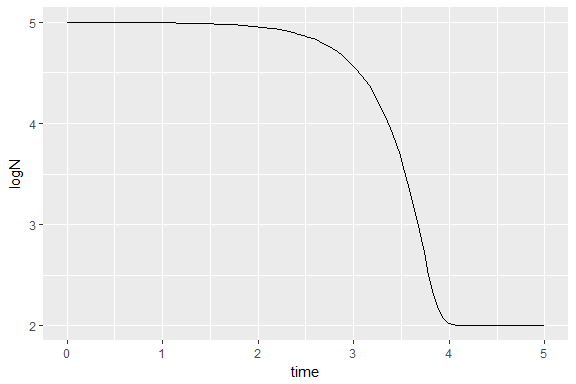Although the effect is not fully clear with the temperature profile used due to the increasing temperature. The model without tail effect can be obtained by assigning zero to N_min. parms_no_tail <- c(D_R = 1, z = 10, N_min = 0, temp_ref = 100, N0 = 100000, C_c0 = 100 ) prediction_no_tail <- predict_inactivation(example_model, times, parms_no_tail, temperature_profile) ## Warning in evalq(log10(N), <environment>): Se han producido NaNs ## Warning in evalq(log10(S), <environment>): Se han producido NaNs plot(prediction_no_tail)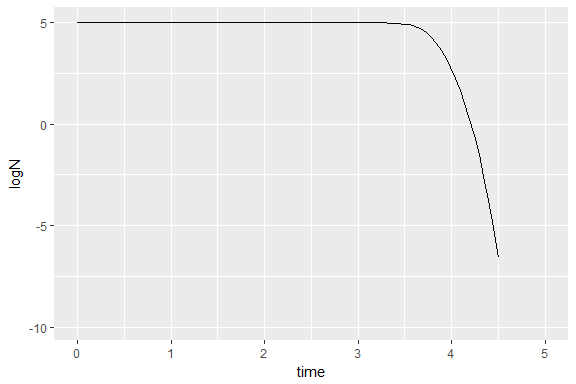Note that this operation causes some warnings. The differential equation of the system is solved for the variable N. For values of this variable very close to zero (of an order of magnitude lower than $$10^{-5}$$), the numerical error may cause the calculated value of N to be lower than zero. Afterwards, when the logarithmic count of microorganisms is calculated, warnings are generated for these values. However, this problem only occurs for values of N below the level of detection in normal laboratory conditions and are not relevant in most cases. The prediction interval can be added to the plot by setting the parameter plot_temp to TRUE. plot(prediction_results, plot_temp = TRUE)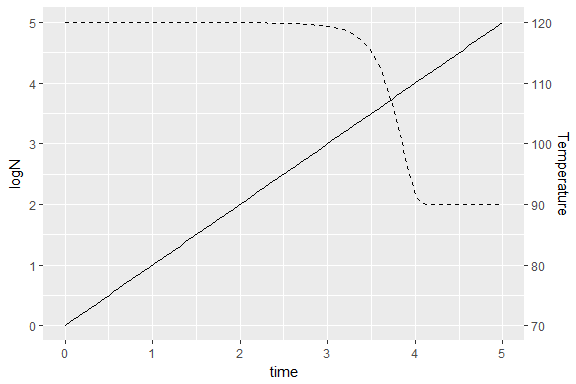If this is the case, the temperature is plotted as a solid line and the prediction curve as a dashed line. ## Fitting of isothermal data The bioinactivation package includes functionality for the fitting of inactivation models to isothermal data. The function fit_isothermal_inactivation() makes use of the nls() function from the stats package to fit the model parameters using non-linear regression. The fit_isothermal_inactivation() function requires the definition of five input parameters: • model_name, • death_data, • starting_point, • known_params and • adjust_log (optional). The experimental data to fit is provided by the argument death_data, which is a data frame. It must contain at least three columns providing the times when the observations were made (time), the temperature of the experiment (temp) and the logarithmic difference observed with respect to the original population (log_diff). The example data set isothermal_inactivation, which already has this format, is used for this demonstration. data(isothermal_inactivation) The model to be used for the adjustment is defined by the character variable model_name. A list of the valid model keys for isothermal adjustment can be obtained by calling the function get_isothermal_model_data() without any arguments. get_isothermal_model_data() ##  "Mafart" "Peleg" "Bigelow" "Arrhenius" Note that Geeraerd’s model is not available for isothermal adjustment. The reason for this is the absence of a secondary model that explains the relation between $$N_{res}$$ and temperature, making impossible the adjustment using non-linear regression. A possible alternative for the adjustment of Geeraerd’s model to individual isothermal experiments is the use of the function fit_dynamic_inactivation() with a constant temperature profile and fixing the value of $$z$$ to an arbitrary value. Bigelow model, whose key is "Bigelow", is used in this example. model_name <- "Bigelow" The names of the parameters of an inactivation model can be obtained by calling the function get_isothermal_model_data() with the model key as argument. Note that for the fitting of isothermal experiments the meta data of the models is gathered calling get_isothermal_model_data() while for making predictions and fitting dynamic experiments get_model_data() is called. model_data <- get_isothermal_model_data(model_name) model_data$params
##  "z"        "D_R"      "temp_ref"

Therefore, the isothermal Bigelow model requires the definition of three parameters: z, D_ref and ref_temp. The fit_isothermal_inactivation() function allows to distinguish between known and unknown (i.e., to be adjusted) model parameters. The known model parameters are set by the input argument known_parameters, which must be a list. The reference temperature is usually fixed to a value close to the experimental ones, we will set it to 100ºC in this example.

known_params = list(temp_ref = 100)

According to the requirements of the nls function, initial points are required for the unknown model parameters. These initial values are provided by the input argument starting_point. This variable needs to be a list with its names identical to those retrieved using get_isothermal_model_data().

starting_point <- list(z = 10,
D_R = 1.5
)

Definition of unrealistic starting points might result in nls raising an error. Hence, it is important to define values relatively close to the solution. This can be done from experience, consulting the literature or testing isothermal predictions with the predict_inactivation() function.

The fit_isothermal_inactivation() functions can base the adjustment on the minimization of the error of either the logarithmic count ($$\mathrm{log}_{10}(N)$$) or the total number of microorganisms ($$N$$). This is controled by the boolean variable adjust_log. In case it is TRUE (default case), the adjustment is based on the error of the logarithmic count. Ohterwise, it is based on the error in the total number of microorganism. In this example, the default option will be used.

The adjustment is made by calling the fit_isothermal_inactivation() function:

iso_fit <- fit_isothermal_inactivation(model_name, isothermal_inactivation,
starting_point, known_params)

This function returns a list of class IsoFitInactivation. This object contains the results of the adjustment, as well as the statistical information of the adjustment process. This information is divided in four entries:

• nls,
• parameters,
• model and
• data.

The information of the adjustment process is provided under the entry nls, where the object returned by the nls function is saved. This argument provides extensive statistical information regarding the adjustment process. For more information, consult the help page for nls.

summary(iso_fit$nls) ## ## Formula: log_diff ~ Bigelow_iso(time, temp, z, D_R, temp_ref) ## ## Parameters: ## Estimate Std. Error t value Pr(>|t|) ## z 7.7605 0.2442 31.78 <2e-16 *** ## D_R 2.9805 0.1422 20.95 <2e-16 *** ## --- ## Signif. codes: 0 '***' 0.001 '**' 0.01 '*' 0.05 '.' 0.1 ' ' 1 ## ## Residual standard error: 0.4801 on 66 degrees of freedom ## ## Number of iterations to convergence: 5 ## Achieved convergence tolerance: 1.126e-06 vcov(iso_fit$nls)  # Calculates variance-covariance matrix {stats}
##               z         D_R
## z    0.05963167 -0.02944040
## D_R -0.02944040  0.02023196
confint(iso_fit$nls) # Calculates confidence intervals {stats} ## Waiting for profiling to be done... ## 2.5% 97.5% ## z 7.285726 8.272271 ## D_R 2.720058 3.296796 The entry parameters contains the values of both the parameters fixed through the input argument known_params and those adjusted by non linear regression. These values are saved as a list. iso_fit$parameters
## $temp_ref ##  100 ## ##$z
##  7.760496
##
## $D_R ##  2.980496 The key of the model used for the adjustment is provided under the header model. iso_fit$model
##  "Bigelow"

The data used for the adjustment is saved under the header data.

head(iso_fit$data) ## time temp log_diff ## 1 0 100 0.0000000 ## 2 1 100 -0.2086205 ## 3 2 100 -0.8500512 ## 4 4 100 -0.8077948 ## 5 6 100 -1.7080181 ## 6 8 100 -2.0522733 The class IsoFitInactivation implements S3 methods for plot(). This method generates a comparison plot between experimental data and predicted values for every single experimental temperature. The title of each of the plots specifies the temperature of the experiments. plot(iso_fit, ylab = "Number of logarithmic reductions", xlab = "Time (min)")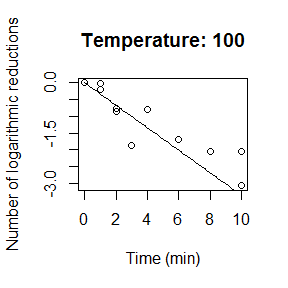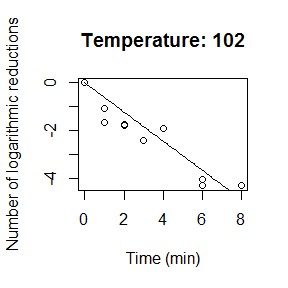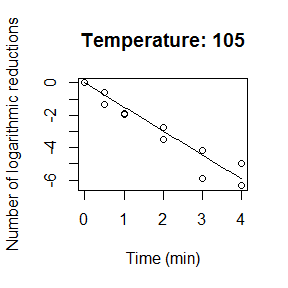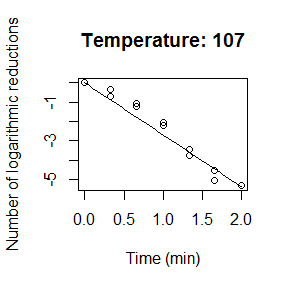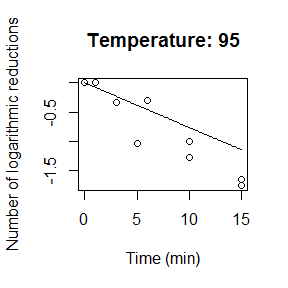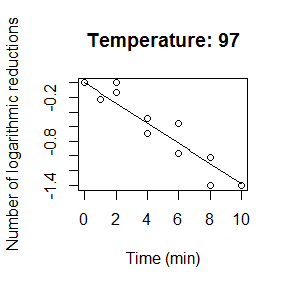The plot can also be generated using ggplot2. To do that, the argument make_gg = TRUE must be added to the call to the plot() function. plot(iso_fit, make_gg = TRUE) + ylab("Number of logarithmic reductions") + xlab("Time (min)")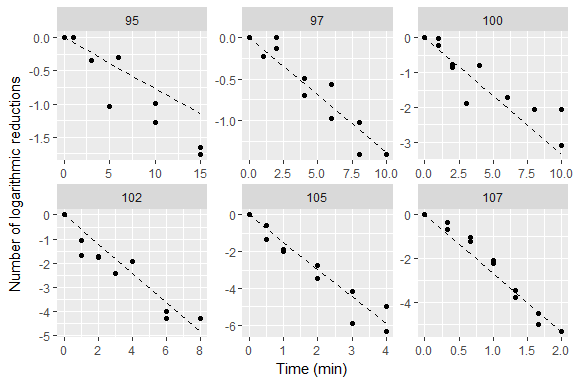## Fitting of dynamic experiments using non-linear regression The bioinactivation package implements the function fit_dynamic_inactivation(), which permits the adjustment of inactivation models to experimental data with time dependent temperature profiles. This is accomplished using the function modFit() of the FME package (Soetaert, Petzoldt, and Setzer 2010b), which adjusts the model using non-linear regression. The function fit_dynamic_inactivation() requires the definition of eight input arguments: • experiment_data, • simulation_model, • temp_profile, • starting_points, • upper_bounds, • lower_bounds, • known_params, • ..., • minimize_log (optional) and • tol0 (optional). The experimental data to fit is provided by the variable experiment_data. It has to be a data frame with at least a column named time, which provides the times when the measurements were taken, and another one named N, with the number of microorganisms counted at each time. The function requires the initial number of microorganism $$N_0$$ to be the same for each experiment. The data set dynamic_inactivation included in the bioinactivation package is used for this demonstration. data(dynamic_inactivation) The inactivation model is specified by the character variable simulation_model. A list of the available models for dynamic adjustment can be obtained by calling the function get_model_data() without any input argument. get_model_data() ##  "Bigelow" "Mafart" "Geeraerd" "Peleg" "Arrhenius" The Weibull-Peleg model is used in this example. simulation_model <- "Peleg" The temperature profile of the experiment is given by the data frame temp_profile, which provides the values of the temperature at discrete time points. Temperatures at time points not included in temp_profile are calculated by linear interpolation. The dynamic_inactivation data set includes the temperature of each observation. Hence, the temperature profile defined by this observations is used for the adjustment. dummy_temp <- data.frame(time = dynamic_inactivation$time,
temperature = dynamic_inactivation$temperature) Although the temperature profile defines several different values of temperature for each time value, only the one calculated by linear interpolation will be used for the resolution of the differential equation. The classification of every degree of freedom of the model (model parameters and initial values of the variables) as either known or to be adjusted is required for the adjustment. The degrees of freedom of the model selected can be obtained by calling get_model_data() with the model key as argument. model_data <- get_model_data(simulation_model) model_data$parameters
##  "k_b"       "temp_crit" "n"
model_data$variables ##  "N" The degrees of freedom (parameters or inial values of variables) known are defined through the named list known_parameters. The names of known model parameters need to be identical to those provided by the function get_model_data(). The names of known initial values must be equal to the variable name provided by get_model_data() plus a caracter zero ("0"). In this example, solely the parameter temp_crit will be considered as known. known_params = c(temp_crit = 100) The rest of the degrees of freedom are adjusted to the experimental data. The fitting algorithm requires the definition of starting points for the adjustment of unknown parameters, as well as upper and lower bounds. This information is passed by the input arguments starting_points, upper_bounds and lower_bounds, which share format with the input argument known_params. In case of unbounded variables, +Inf or -Inf must be assigned to the desired entries of upper_bounds or lower_bounds. starting_points <- c(n = 1, k_b = 0.25, N0 = 1e+05) upper_bounds <- c(n = 2, k_b = 1, N0 = Inf) lower_bounds <- c(n = 0, k_b = 0, N0 = 1e4) Unrealistic initial points for the adjustment will cause the fitting algorithm to fail. Realistic initial points can be obtained from experience, literature or by testing different predictions using predict_inactivation(). It is advisable to use reasonable lower and upper bounds, so degrees of freedom do not become negative or unrealistically large. The adjustment can be based on the minimization of the error of the total count of microorganism ($$N$$) or on the logarithmic count ($$\mathrm{log}_{10}N$$). This distinction is made by the boolean input argument minimize_log. In case it is TRUE, the error of the logarithmic count is minimized. By default, this parameter is set to TRUE. It will not be modified. The inactivation models based on the Weibull distribution are singular for $$t=0$$. For this reason, every value of time in times lower than the optional argument tol0 is changed for tol0. By default, tol0 = 1e-05. Further arguments can be passed to the modFit() function through the ... argument. The adjustment is made by calling the function fit_dynamic_inactivation(): dynamic_fit <- fit_dynamic_inactivation(dynamic_inactivation, simulation_model, dummy_temp, starting_points, upper_bounds, lower_bounds, known_params) The function returns a list of class FitInactivation with three entries: • fit_results, • data and • best_prediction. fit_results provides the instance of modFit returned by the modFit() function. This variable contains broad information regarding the adjustment process. For more information, refer to the help page of the function. summary(dynamic_fit$fit_results)
##
## Parameters:
##      Estimate Std. Error t value Pr(>|t|)
## n   3.929e-01  9.163e-02   4.288 0.000124 ***
## k_b 7.273e-01  3.212e-02  22.645  < 2e-16 ***
## N0  1.189e+05  3.139e+04   3.788 0.000541 ***
## ---
## Signif. codes:  0 '***' 0.001 '**' 0.01 '*' 0.05 '.' 0.1 ' ' 1
##
## Residual standard error: 0.4375 on 37 degrees of freedom
##
## Parameter correlation:
##           n     k_b      N0
## n    1.0000 -0.1682 -0.4349
## k_b -0.1682  1.0000  0.7321
## N0  -0.4349  0.7321  1.0000

The header data contains a data frame with the experimental data used for the fit.

head(dynamic_fit$data) ## time logN ## 1 0.00001 5.025306 ## 2 0.37000 5.110590 ## 3 0.74000 5.037426 ## 4 1.11000 4.832509 ## 5 1.30000 4.255273 ## 6 1.80000 2.602060 Under best_prediction, an instance SimulInactivation with the results of the best prediction is saved. For more information about this instance, refer to the documentation of predict_inactivation(). The parameters of the model calculated are saved under the entry model_parameters of the SimulInactivation instance. dynamic_fit$best_prediction$model_parameters ##$n
##  0.3929265
##
## $k_b ##  0.72728 ## ##$N0
##  118901.6
##
## $temp_crit ##  100 The FitInactivation object includes S3 methods for the creation of a comparison plot between the experimental results and the prediction made by the model parameters adjusted. Two different versions of this function are implemented, one in ggplot2 (default) and another one in base R. By default, the plot ggplot2 implementation is called, which returns an object of class ggplotwith the graphic generated. This allows for further editting: p <- plot(dynamic_fit) p + theme_light()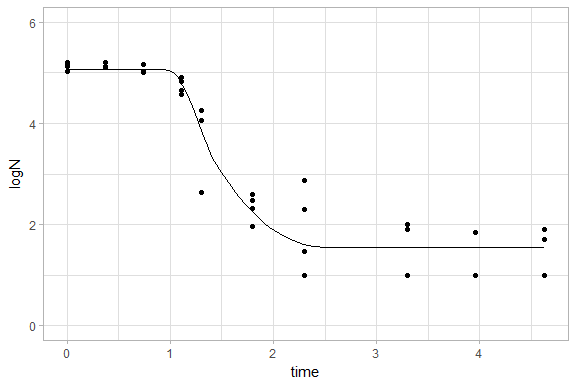The temperature profile can be added to the plot by setting the argument plot_temp to TRUE. plot(dynamic_fit, plot_temp = TRUE)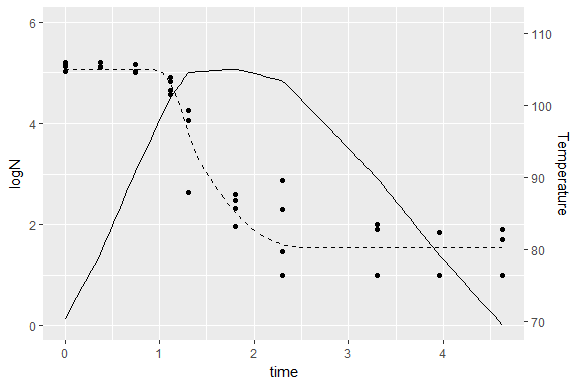In this case, the temperature profile is plotted as a solid line and the survivor curve as a dashed line. The base R graph can be generated including the argument make_gg = FALSE. plot(dynamic_fit, make_gg = FALSE, xlab = "Time (min)", ylab = "logN")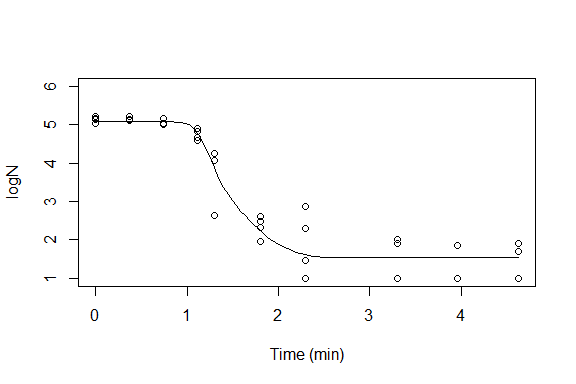## Fitting of dynamic experiments using Markov Chain Monte Carlo methods The function fit_inactivation_MCMC() is able to wrap the modMCMC() function from the FME package (Soetaert, Petzoldt, and Setzer 2010b). Therefore, inactivation models can be adjusted to dynamic experiments using a Markov Chain Monte Carlo algorithm. The function fit_inactivation_MCMC() has ten input arguments: • experiment_data, • simulation_model, • temp_profile, • starting_points, • upper_bounds, • lower_bounds, • known_params, • ..., • minimize_log and • tol0. Every input argument except for ... are identical in meaning and format to those defined for fit_dynamic_inactivation(), enabling for easy switch between both adjustment methods. The only differents is that, in this case, the ... arguments allows to send further arguments to the modMCMC() function. In order to highlight the similitudes between this function and fit_dynamic_inactivation(), the input arguments shared by both functions will be reused in this example. An additional argument will be passed to the modMCMC() function, the niter argument. This argument defines the number of iterations of the Markov Chain Monte Carlo algorithm for the adjustment. set.seed(82619) MCMC_fit <- fit_inactivation_MCMC(dynamic_inactivation, simulation_model, dummy_temp, starting_points, upper_bounds, lower_bounds, known_params, niter = 50) ## number of accepted runs: 36 out of 50 (72%) fit_inactivation_MCMC() returns a list of class FitInactivationMCMC with three entries: • modMCMC, • best_prediction and • data. modMCMC provides the instance of modMCMC returned by the function modMCMC(). This object includes broad information about the adjustment process. For more information, refer to the help of modMCMC(). summary(MCMC_fit$modMCMC)
##              n        k_b        N0
## mean 1.4063525 0.21161020  61562.91
## sd   0.1580651 0.03826561  22560.25
## min  1.0000000 0.14551260  17193.30
## max  1.6319618 0.27929880 100704.73
## q025 1.3338920 0.18414717  48844.96
## q050 1.4507580 0.20366414  57630.62
## q075 1.5045636 0.23767184  80243.34

best_prediction contains a list of class SimulInactivation with the prediction of the adjusted parameters. This object has already been described in the description of the function predict_inactivation(). Finally, the data used for the fit is saved under data.

The FitInactivationMCMC instance includes a S3 implementation of plot() for comparing the prediction against the data. Two different versions of this function are implemented, one in ggplot2 (default) and another one in base R. The default version returns an instance of ggplot with the graphic generated, allowing further edition.

p <- plot(MCMC_fit)
p + xlab("Time (min)")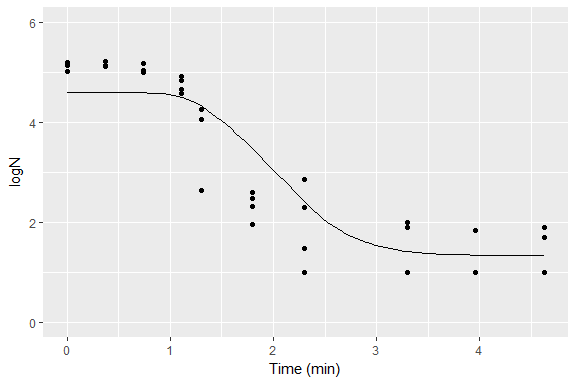The temperature profile can be added to the plot by setting the argument plot_temp to TRUE.

plot(MCMC_fit, plot_temp = TRUE)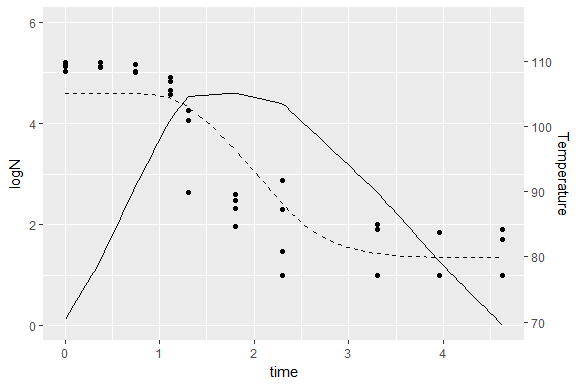If this is the case, the temperature profile is shown as a solid line and the survivor curve as a dashed line.

The base R graph can be generated including the argument make_gg = FALSE.

plot(MCMC_fit, make_gg = FALSE,
xlab = "Time (min)", ylab = "logN")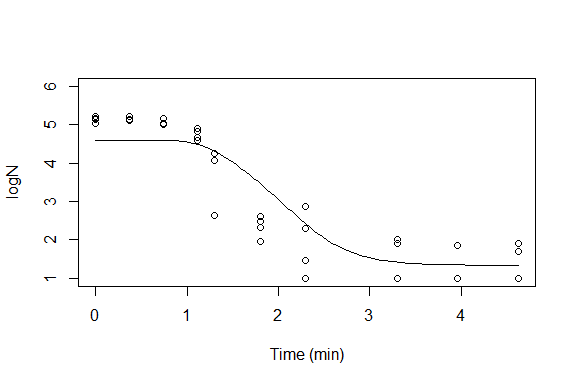## Generation of prediction intervals using Monte Carlo methods

bioinactivation includes the function predict_inactivation_MCMC(), which allows the generation of prediction intervals following a Monte Carlo approach. This function has six input arguments, four of which are optional:

• fit_object,
• temp_profile,
• n_simulations (optional),
• times (optional),
• quantiles (optional) and
• additional_pars (optional).

The argument fit_object supplies the fit object to use for the genration of the prediction interval. The function is able to generate prediction intervals from objects of classes IsoFitInactivation, FitInactivation and FitInactivationMCMC. The algorithm for the generation of the prediction interval differs if the model was fitted using non-linear regression (IsoFitInactivation and FitInactivation) or MCMC (FitInactivationMCMC).

On every case, the algorithm is based on a random sampling of the model parameters adjusted. For objects of classes IsoFitInactivation and FitInactivation it is considered that the model parameters follow a multivariate normal distribution. On the other hand, the sampling of objects of class FitInactivationMCMC is based on the iterations performed by the modMCMC() function. One example will be presented for each one of this three cases.

The object dynamic_fit of class FitInactivation will be used first. The modFit() function provides estimates of the model parameters as well as an estimation of the unscaled covariance matrix. The predict_inactivation_MCMC() function generates a random sampling of the model parameters using the mvrnorm function from the MASS package (Venables and Ripley, 2002).

library(MASS)
sP <- summary(dynamic_fit)
sample_iso <- mvrnorm(1000, coef(dynamic_fit$fit_results), sP$cov.unscaled)

ggplot(as.data.frame(sample_iso)) +
geom_point(aes(x = N0, y = k_b), alpha = 0.5, size = 3) +
ggtitle("Example of multivariate normal sampling")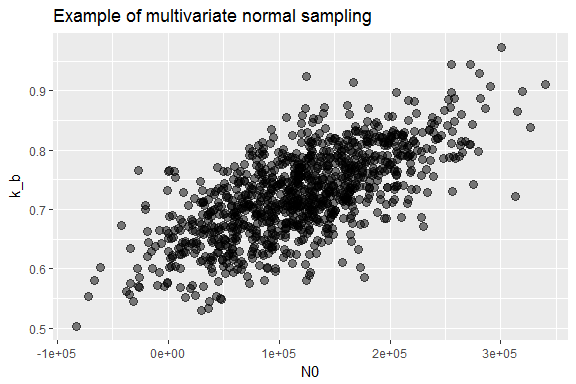Due to the hypothesis used, the sampling may produce negative values of the model parameters, producing spurious results. Samples with negative values of the model parameters are omitted.

The temperature profile to use for the prediction is defined by the argument temp_profile. It must be a data frame with one column named time and another one named temperature.

dummy_temp <- data.frame(time = c(0, 1, 4, 6), temperature = c(80, 110, 110, 80))
ggplot(dummy_temp) +
geom_line(aes(x = time, y = temperature)) +
ggtitle("Temperature profile used for the prediction interval")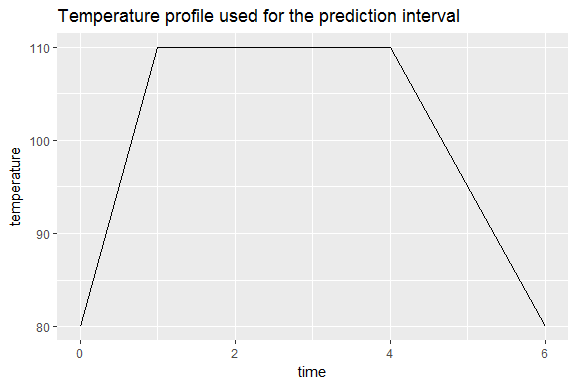The optional arugment n_simulations is an integer which defines the number of Monte Carlo predictions to be made for the generation of the prediction interval. The higher the uncertainty in the model parameters, the larger this argument must be to achieve convergence. By default, n_simulations = 100.

The optional argument times is a numeric vector which defines the time points at which the solution is calculated. The larger the length of this vector, the longer the computation time. On the other hand, if the length of this vector is excessively low, a rough prediction interval will be generated. By default, the time points used for the model fit will be reused.

The generation of the prediction interval is based on the quantiles of the Monte Carlo simulations at individual time points. The quantiles to calculate are defined by the optional argument quantiles, which is a numeric vector. By default, this argument is set to quantiles = c(2.5, 97.5) defining a prediction interval with at 0.95 confidence level. In case quantiles = NULL, no statistics are calculated and the results of all the Monte Carlo simulations are returned.

The initial number of microorganism is not required for the adjustment of isothermal experiments. However, this parameter is required for the generation of prediction intervals. The argument additional_pars allows to include additional arguments originally not present in the model as a named numeric list.

Firstly, a prediction interval will be generated from the dynamic_fit object with just the two mandatory arguments.

set.seed(7163)
pred_MCMC_1 <- predict_inactivation_MCMC(dynamic_fit, dummy_temp)
head(pred_MCMC_1)
##       times     mean   median     2.5%    97.5%
## 1 0.0000000 122588.2 122997.6 15378.05 249840.6
## 2 0.0944898 122588.0 122997.1 15377.99 249840.5
## 3 0.1889796 122586.0 122993.7 15377.71 249837.5
## 4 0.2834694 122571.1 122973.5 15376.28 249808.6
## 5 0.3779592 122461.2 122850.2 15368.80 249552.0
## 6 0.4724490 121629.3 122093.6 15327.28 247293.9

A data frame of class PredInactivationMCMC is returned. This object includes the mean and the median of the Monte Carlo simulations at each time point requested. Moreover, it includes the 0.025 and 0.975 quantiles defining a 0.95 prediction intervals. This object includes an S3 method for plot() which allows to visualize the prediction interval generated.

p <- plot(pred_MCMC_1)
p + xlab("time")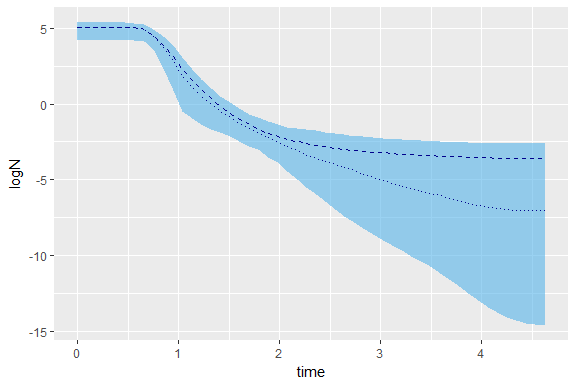This plot shows the mean of the simulations as a dashed line, its median as a pointed line and the prediction interval generated as a blue ribbon. The differences in the mean and median by the end of the experiment suggest that the solutions are not normally distributed.

The disperssion of the Monte Carlo simulations can be observed including the argument quantiles = NULL. Note that the ggplot2 plot is not compatible with PredInactivationMCMC without the statistics calculated. On this case, the base plot system has to be used including the make_gg = FALSE argument in the call to the plot() function.

set.seed(7163)
pred_MCMC_2 <- predict_inactivation_MCMC(dynamic_fit, dummy_temp, quantiles = NULL)
plot(pred_MCMC_2, make_gg = FALSE,
xlab = "Time (min)", ylab = "logN")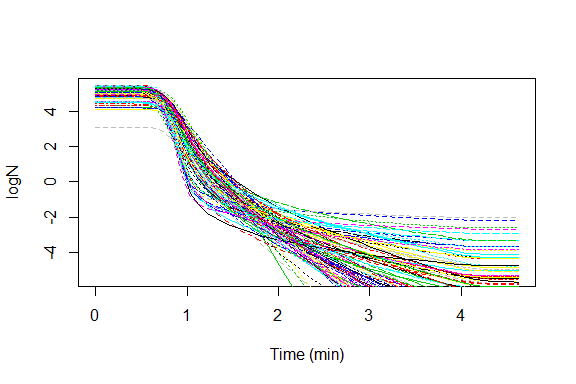The results show that a larger number of iterations of the Monte Carlo algorithm may be needed for the prediction interval to converge. Therefore, the n_simulations argument will be increased.

set.seed(7163)
pred_MCMC_3 <- predict_inactivation_MCMC(dynamic_fit, dummy_temp, n_simulations = 200)
p <- plot(pred_MCMC_3)
p + ylab("logN")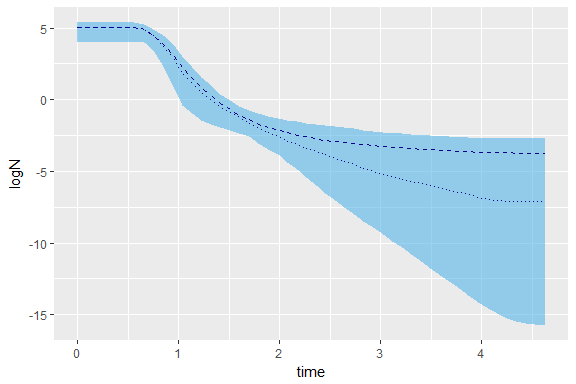The function predict_inactivation_MCMC() allows the generation of assymetrical prediction intervals. In food microbiology, the more resistant microorganism are usually more relevant than the most weaks. A one-sided prediction interval can be generated by defining quantiles = c(0, 95).

set.seed(7163)
pred_MCMC_4 <- predict_inactivation_MCMC(dynamic_fit, dummy_temp, quantiles = c(0, 95))
p <- plot(pred_MCMC_4)
p + ylab("logN")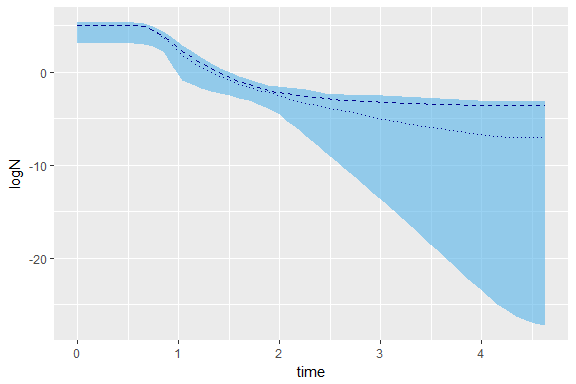The sampling generated for objects of class iso_fit follows the same hypothesis presented for instances of FitInactivation. The nls object provides estimates of the model parameters as well as an estimation of the model covariances. The predict_inactivation_MCMC() function generates a random sampling of the model parameters using the mvrnorm function from the MASS package (Venables and Ripley, 2002).

library(MASS)
sample_iso <- mvrnorm(1000, coef(iso_fit$nls), vcov(iso_fit$nls))

As already mentioned, the initial number of microorganisms is not needed for the performance of isothermal model adjustment. Nevertheless, this argument is required for the generation of prediction intervals. Therefore, the initial number of microorganisms must be passed to the function through the additional_pars argument.

set.seed(918734)
times <- seq(0, 6, length = 50)
dummy_temp <- data.frame(time = c(0, 3, 4, 6), temperature = c(80, 110, 110, 80))
pred_int_iso <- predict_inactivation_MCMC(iso_fit, dummy_temp,
times = times,
p <- plot(pred_int_iso)
p + xlab("time")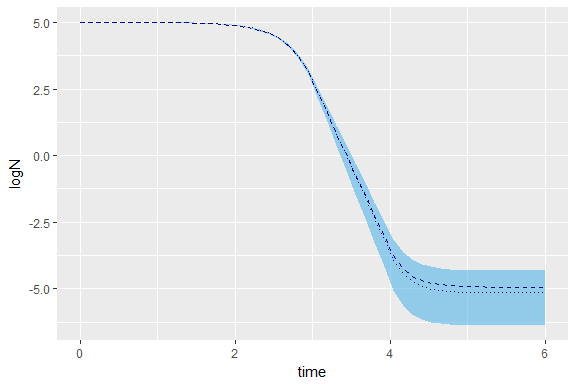The instances of FitInactivationMCMC save the values of the model parameters used during the iterations of the Monte Carlo algorithm. Hence, instead of redoing the sampling, this values are reused for the generation of the prediction interval. The adventage of this method in comparison with the one used for models adjusted using non-linear regression is the omission of any hypothesis regarding the probability distribution of the model parameters.

Appart from this difference in the hypothesis, the call to the function predict_inactivation_MCMC() is performed in the same way as for instances of FitInactivation.

set.seed(918734)
dummy_temp <- data.frame(time = c(0, 1, 4, 6), temperature = c(80, 110, 110, 80))
pred_int_MCMC <- predict_inactivation_MCMC(MCMC_fit, dummy_temp)
p <- plot(pred_int_MCMC)
p + xlab("time")